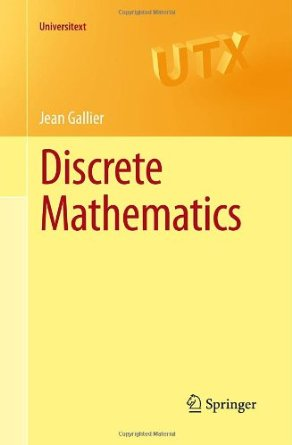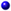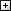Processing ......FreeComputerBooks.com Free Computer, Mathematics, Technical Books and Lecture Notes, etc.

Discrete Mathematics for Computer Science
Want to know the elevation (AMSL) at any spot all over world? Click here for details.
• Title Discrete Mathematics for Computer Science
• Author(s) Jean H. Gallier
• Publisher: Springer; 2011 edition (January 25, 2011)
• Hardcover/Paperback 477 pages
• eBook PDF, PostScript, and other formats
• Language: English
• ASIN: 1441980466
• ISBN-13: 978-1441980465Book Description

This book gives an introduction to discrete mathematics for beginning undergraduates. One of original features of this book is that it begins with a presentation of the rules of logic as used in mathematics. Many examples of formal and informal proofs are given.

With this logical framework firmly in place, the book describes the major axioms of set theory and introduces the natural numbers. The rest of the book is more standard. It deals with functions and relations, directed and undirected graphs, and an introduction to combinatorics.

There is a section on public key cryptography and RSA, with complete proofs of Fermat's little theorem and the correctness of the RSA scheme, as well as explicit algorithms to perform modular arithmetic. The last chapter provides more graph theory. Eulerian and Hamiltonian cycles are discussed. Then, we study flows and tensions and state and prove the max flow min-cut theorem.

We also discuss matchings, covering, bipartite graphs.

The book is highly illustrated and each chapter ends with a list of problems of varying difficulty. Undergraduates in mathematics and computer science will find this book useful.

Reviews, Ratings, and Recommendations: Related Book Categories: Read and Download Links:Similar Books:All CategoriesRecent BooksIT Research LibraryMiscellaneous BooksComputer LanguagesComputer ScienceData Science/DatabasesElectronic EngineeringJava and Java EE (J2EE)Linux and UnixMathematicsMicrosoft and .NETMobile ComputingNetworking and CommunicationsSoftware EngineeringSpecial TopicsWeb Programming